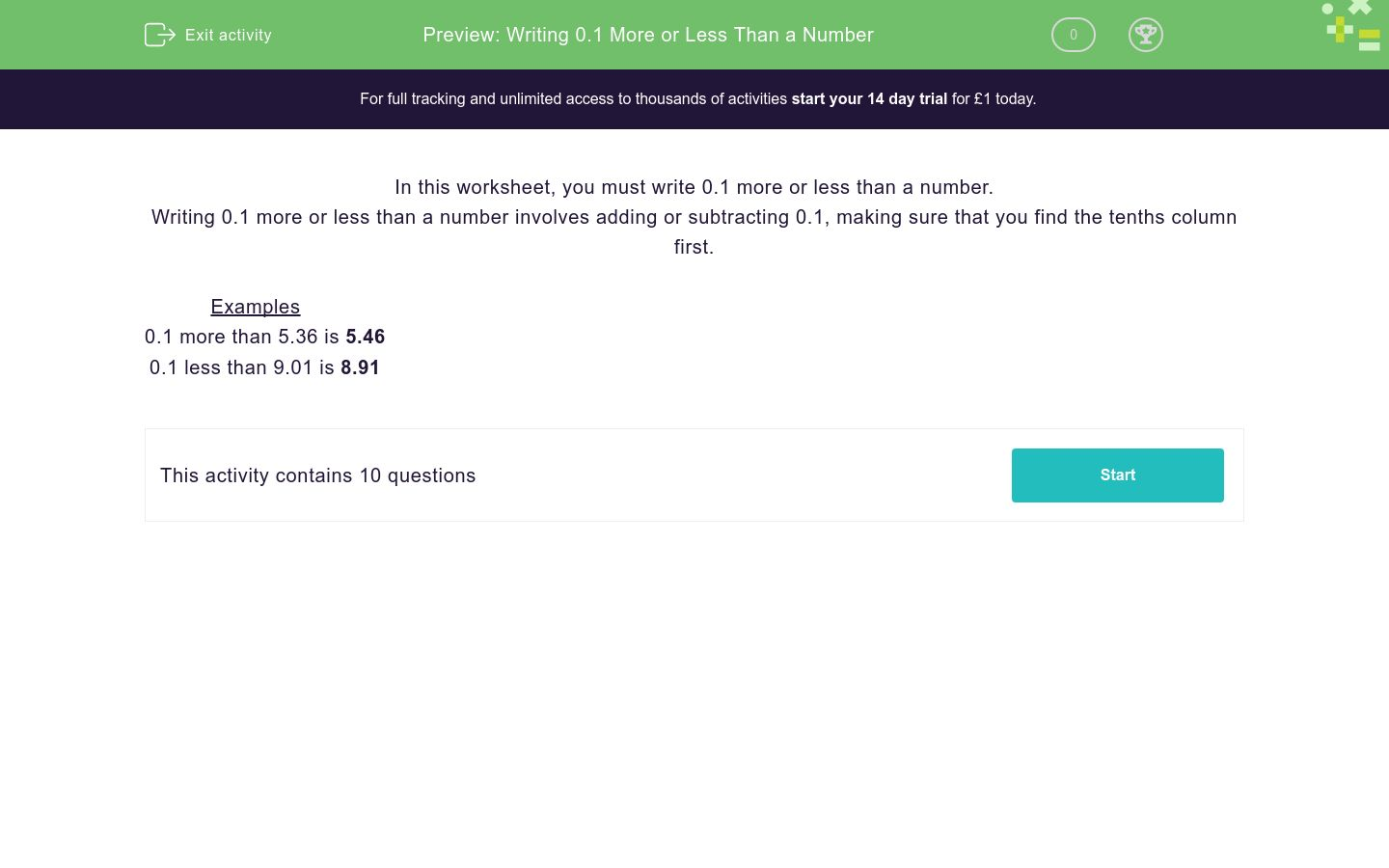# Writing 0.1 More or Less Than a Number

In this worksheet, students add or subtract 0.1 to or from a number.Key stage:  KS 3

Curriculum topic:   Number

Curriculum subtopic:   Use Place Value for All Numbers

Difficulty level:### QUESTION 1 of 10

In this worksheet, you must write 0.1 more or less than a number.

Writing 0.1 more or less than a number involves adding or subtracting 0.1, making sure that you find the tenths column first.

Examples

0.1 more than 5.36 is 5.46

0.1 less than 9.01 is 8.91

What is 0.1 more than 4.92? ________

What is 0.1 less than 5.94? ________

What is 0.1 more than 2.1? ________

What is 0.1 less than 1.75? ________

What is 0.1 more than 5.05? ________

What is 0.1 less than 4.26? ________

What is 0.1 more than 8.14? ________

What is 0.1 less than 8.57? ________

What is 0.1 more than 7.93? ________

What is 0.1 less than 4.06? ________

• Question 1

What is 0.1 more than 4.92? ________

5.02
• Question 2

What is 0.1 less than 5.94? ________

5.84
• Question 3

What is 0.1 more than 2.1? ________

2.2
• Question 4

What is 0.1 less than 1.75? ________

1.65
• Question 5

What is 0.1 more than 5.05? ________

5.15
• Question 6

What is 0.1 less than 4.26? ________

4.16
• Question 7

What is 0.1 more than 8.14? ________

8.24
• Question 8

What is 0.1 less than 8.57? ________

8.47
• Question 9

What is 0.1 more than 7.93? ________

8.03
• Question 10

What is 0.1 less than 4.06? ________

3.96
---- OR ----

Sign up for a £1 trial so you can track and measure your child's progress on this activity.

### What is EdPlace?

We're your National Curriculum aligned online education content provider helping each child succeed in English, maths and science from year 1 to GCSE. With an EdPlace account you’ll be able to track and measure progress, helping each child achieve their best. We build confidence and attainment by personalising each child’s learning at a level that suits them.

Get started